### 2Constructors

The examples in this section are easiest to compare when using an auto camera that doesn’t depend on the Pict3D instance.
 > (current-pict3d-auto-camera (λ (_) (point-at (pos 1 1 1) origin)))

 type
 predicate
The type and predicate for 3D scenes.

 type
 procedure(combine p ...) → Pict3D p : Pict3Ds
A Pict3Ds instance is either a Pict3D or a list of Pict3Ds; i.e. a tree.

The combine function returns a new Pict3D that contains all of Pict3D instances in all of its arguments p .... Usually, the order they’re given in doesn’t affect the result. When it does, don’t rely on any particular order.

 value
 procedure p : Pict3D
A Pict3D containing nothing, and a function to detect empty Pict3Ds.

procedure

 (rectangle corner1 corner2 [ #:inside? inside?]) → Pict3D
corner1 : Pos
corner2 : Pos
inside? : Any = #f
(rectangle center scale [#:inside? inside?])  Pict3D
center : Pos
scale : (U Dir Real)
inside? : Any = #f
(cube center scale [#:inside? inside?])  Pict3D
center : Pos
scale : Real
inside? : Any = #f
The first form returns a Pict3D containing a single rectangle with corners corner1 and corner2. The corners may be any pair that are the opposite endpoints of a main diagonal.

Example:
 > (rectangle origin (pos 1/2 1/2 1/2))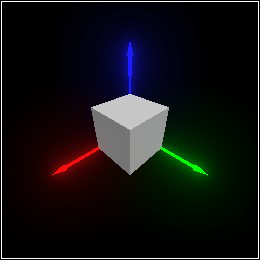If the second argument is a direction vector or a scale, the first argument is regarded as a center point. If scale is a direction, the components are interpreted as axis-aligned half-widths.

Example:
 > (rectangle origin (dir 1/4 1/2 3/4))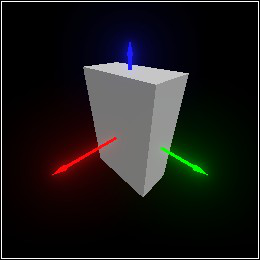When scale is a real number, (rectangle center scale) is equivalent to (rectangle center (dir scale scale scale)), and (cube center scale) is equivalent to (rectangle center scale).

When inside? is non-#f, the rectangle’s surfaces face inward.

Examples:
> (rectangle origin (pos 1.25 1.25 1.25) #:inside? #t)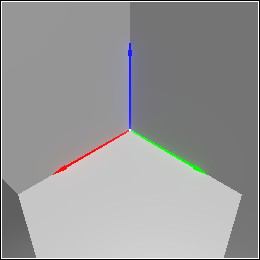> (deform (tessellate (combine (with-color (rgba "firebrick") (combine (rectangle (pos 0 0 5/8) (dir 1/2 1/2 1/8)) (rectangle (pos 0 0 -5/8) (dir 1/2 1/2 1/8)))) (rectangle origin (dir 1/2 1/2 1/2)))) (twist 120))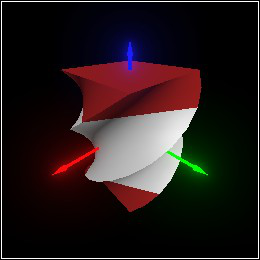procedure

 (ellipsoid corner1 corner2 [ #:inside? inside?]) → Pict3D
corner1 : Pos
corner2 : Pos
inside? : Any = #f
(ellipsoid center scale [#:inside? inside?])  Pict3D
center : Pos
scale : (U Dir Real)
inside? : Any = #f
(sphere center radius [#:inside? inside?])  Pict3D
center : Pos
inside? : Any = #f
Returns a Pict3D containing the largest ellipsoid that fits inside (rectangle corner1 corner2) or (rectangle center scale).

Examples:
 > (combine (ellipsoid origin (pos 1/2 1/2 1/2)) (with-color (rgba "red" 0.5) (rectangle origin (pos 1/2 1/2 1/2))))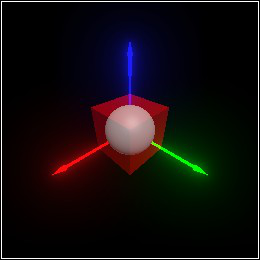> (combine (ellipsoid origin (dir 1/4 1/2 3/4)) (with-color (rgba "red" 0.5) (rectangle origin (dir 1/4 1/2 3/4))))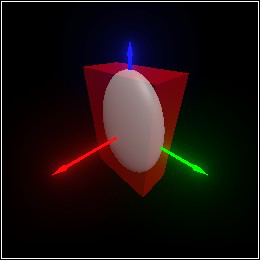As with cube and rectangle, (sphere center radius) is equivalent to (ellipsoid center radius).

Example:
 > (adaptive-deform (with-color (rgba "turquoise") (ellipsoid (pos 0 -1/2 0) (dir 1/4 1/4 1))) (twist 360))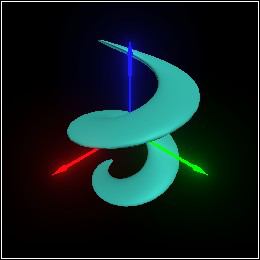When inside? is non-#f, the ellipsoid surface faces inward. (See rectangle.)

procedure

 (cylinder corner1 corner2 [ #:inside? inside? #:arc arc #:top-cap? top-cap? #:bottom-cap? bottom-cap? #:start-cap? start-cap? #:end-cap? end-cap? #:outer-wall? outer-wall?]) → Pict3D
corner1 : Pos
corner2 : Pos
inside? : Any = #f
arc : Arc = circle-arc
top-cap? : Any = #t
bottom-cap? : Any = #t
start-cap? : Any = #t
end-cap? : Any = #t
outer-wall? : Any = #t
 (cylinder center scale #:  ...) → Pict3D
center : Pos
scale : (U Dir Real)
<cylinder-keyword> : <cylinder-keyword-type>
Returns a Pict3D containing the largest vertical cylinder that fits inside (rectangle corner1 corner2) or (rectangle center scale).

Examples:
 > (combine (cylinder origin (pos 1/2 1/2 1/2)) (with-color (rgba "red" 0.5) (rectangle origin (pos 1/2 1/2 1/2))))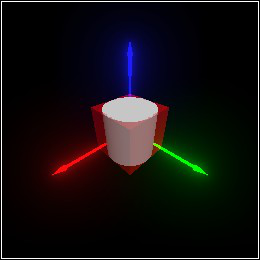> (combine (cylinder origin (dir 1/4 1/2 3/4)) (with-color (rgba "red" 0.5) (rectangle origin (dir 1/4 1/2 3/4))))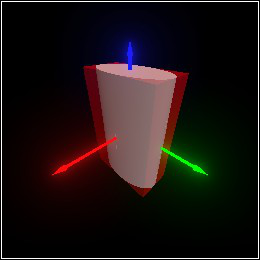When inside? is non-#f, the cylinder’s surfaces face inward. (See rectangle.) The remaining boolean arguments determine which parts of the cylinder’s surface are created.

The arc argument determines the start and end angle swept out by the vertical cap to create the cylinder.

Examples:
> (cylinder origin 1/2 #:arc (arc 90 360))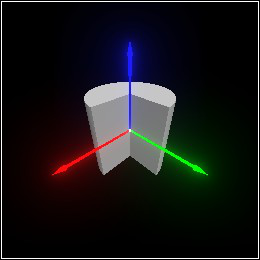> (move (bend (combine (tessellate (with-color (rgba "lightsteelblue") (cylinder (pos 0 0 1) (dir 1/4 1/4 1) #:arc (arc -90 90))) #:max-edge 1/12) (with-color (rgba "slategray") (combine (rectangle (pos 1/8 0 -1/8) (dir 3/16 5/16 1/8)) (rectangle (pos 1/8 0 (+ 2 1/8)) (dir 3/16 5/16 1/8))))) -180 (interval 0 2)) (dir (/ 2 pi) 0 1/4))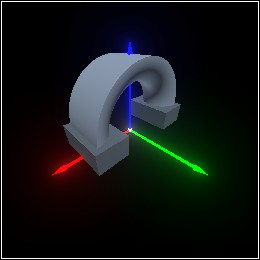procedure

 (cone corner1 corner2 [ #:inside? inside? #:arc arc #:bottom-cap? bottom-cap? #:start-cap? start-cap? #:end-cap? end-cap? #:outer-wall? outer-wall?]) → Pict3D
corner1 : Pos
corner2 : Pos
inside? : Any = #f
arc : Arc = circle-arc
bottom-cap? : Any = #t
start-cap? : Any = #t
end-cap? : Any = #t
outer-wall? : Any = #t
 (cone center scale #:  ...) → Pict3D
center : Pos
scale : (U Dir Real)
<cone-keyword> : <cone-keyword-type>
Returns a Pict3D containing the largest upward-pointing cone that fits inside (rectangle corner1 corner2) or (rectangle center scale).

Examples:
 > (combine (cone origin (pos 1/2 1/2 1/2)) (with-color (rgba "red" 0.5) (rectangle origin (pos 1/2 1/2 1/2))))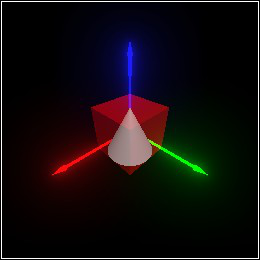> (combine (cone origin (dir 1/4 1/2 3/4)) (with-color (rgba "red" 0.5) (rectangle origin (dir 1/4 1/2 3/4))))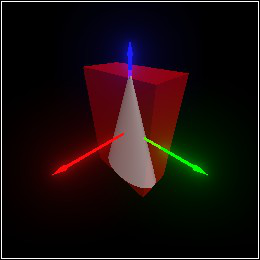When inside? is non-#f, the cone’s surfaces face inward. (See rectangle.) The remaining boolean arguments determine which parts of the cone’s surface are created.

The arc argument determines the start and end angle swept out by the triangular cap to create the cone.

Example:
 > (cone origin 1/2 #:arc (arc 90 360))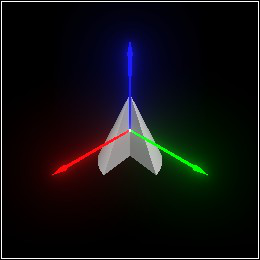procedure

 (pipe corner1 corner2 [ #:inside? inside? #:arc arc #:bottom-radii bottom-radii #:top-radii top-radii #:top-cap? top-cap? #:bottom-cap? bottom-cap? #:start-cap? start-cap? #:end-cap? end-cap? #:inner-wall? inner-wall? #:outer-wall? outer-wall?]) → Pict3D
corner1 : Pos
corner2 : Pos
inside? : Any = #f
arc : Arc = circle-arc
bottom-radii : Interval = (interval 1/2 1)
top-cap? : Any = #t
bottom-cap? : Any = #t
start-cap? : Any = #t
end-cap? : Any = #t
inner-wall? : Any = #t
outer-wall? : Any = #t
 (pipe center scale #:  ...) → Pict3D
center : Pos
scale : (U Dir Real)
<pipe-keyword> : <pipe-keyword-type>
Returns a Pict3D containing the largest vertical, hollow cylinder that fits inside (rectangle corner1 corner2) or (rectangle center scale).

Examples:
 > (combine (pipe origin (pos 1/2 1/2 1/2)) (with-color (rgba "red" 0.5) (rectangle origin (pos 1/2 1/2 1/2))))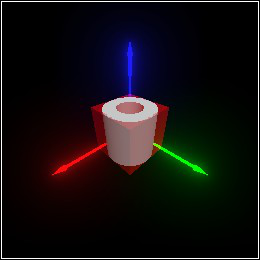> (combine (pipe origin (dir 1/4 1/2 3/4)) (with-color (rgba "red" 0.5) (rectangle origin (dir 1/4 1/2 3/4))))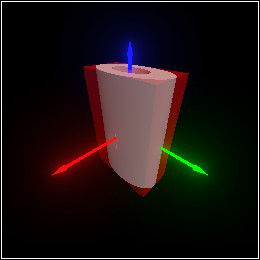When inside? is non-#f, the pipe’s surfaces face inward. (See rectangle.) The remaining boolean arguments determine which parts of the pipe’s surface are created.

The arc argument determines the start and end angle swept out by a trapezoid to create the pipe.

Example:
 > (pipe origin 1/2 #:arc (arc 90 360))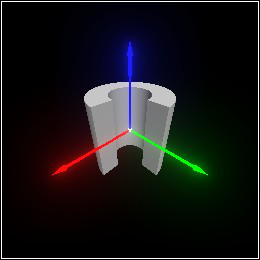The bottom-radii and top-radii intervals give the fractional radius of the inner and outer wall, on the top and bottom of the pipe.

Example:
 > (pipe origin 3/4 #:arc (arc 90 0) #:top-radii (interval 1/2 5/8) #:bottom-radii (interval 1/4 1))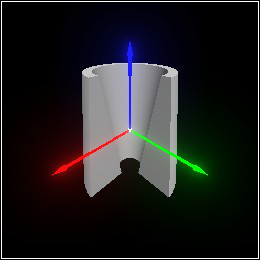The fractional radii may exceed 1.

Example:
 > (let* ([a  (arc 135 45)] [p  (with-color (rgba "crimson") (pipe origin (dir 1/2 1/2 1/16) #:arc a #:bottom-radii (interval 7/8 9/8)))]) (deform (tessellate (combine (move-z p -11/16) (move-z p 11/16) (with-color (rgba "lavender") (pipe origin (dir 1/2 1/2 5/8) #:arc a #:bottom-radii (interval 7/8 1))))) (bend 45 (interval -1/2 1/2))))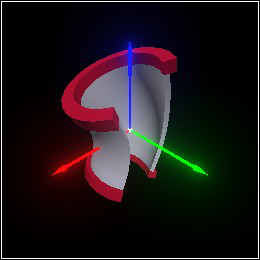procedure

 (ring corner1 corner2 [ #:back? back? #:arc arc #:radii radii]) → Pict3D
corner1 : Pos
corner2 : Pos
back? : Any = #f
arc : Arc = circle-arc
 (ring center scale #:  ...) → Pict3D
center : Pos
scale : (U Dir Real)
<ring-keyword> : <ring-keyword-type>
Returns a Pict3D containing a disk or a ring, placed vertically in the center of the rectangle defined by corner1 and corner2, or by center and scale.

Examples:
 > (combine (ring origin (pos 1/2 1/2 1/2)) (with-color (rgba "red" 0.5) (rectangle origin (pos 1/2 1/2 1/2))))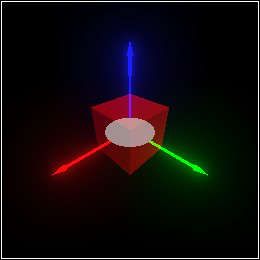> (combine (ring origin (dir 1/4 1/2 3/4)) (with-color (rgba "red" 0.5) (rectangle origin (dir 1/4 1/2 3/4))))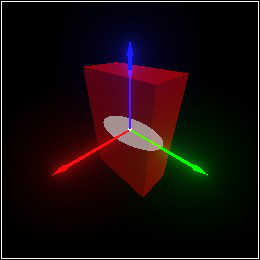When back? is non-#f, the ring surface faces downward.

The arc argument determine the start and end angle swept out by a line to create the ring.

The radii interval argument gives the fractional radii of the inner and outer circle.

Example:
 > (deform (tessellate (combine (ring origin 2 #:arc (arc 90 360) #:radii (interval 3/4 1)) (with-color (rgba 1 1/4 1/4) (ring origin 2 #:arc (arc 90 360) #:radii (interval 1/2 3/4))) (with-color (rgba 1/4 1/2 1) (ring origin 2 #:arc (arc 90 360) #:radii (interval 1/4 1/2)))) #:max-edge 1/8 #:max-angle 5) (displace (λ (x y) (* 1/8 (+ (sin (* 4 (atan y x))) (sin (* pi (+ (sqr x) (sqr y)))))))))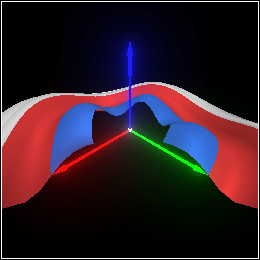procedure

 (triangle corner1 corner2 corner3 [ #:back? back?]) → Pict3D
corner1 : (U Pos Vertex)
corner2 : (U Pos Vertex)
corner3 : (U Pos Vertex)
back? : Any = #f
Returns a Pict3D containing a triangle with the given corners. By default, the triangle is visible only from viewpoints for which its corners appear to be in counterclockwise order. When back? is #t, it’s visible only from viewpoints for which its corners appear to be in clockwise order.

A corner may be either a position vector or a vertex. When a corner is a Pos instance, its normal is that of the plane the triangle lies in, and its reflected color, emitted color, and material are the values of current-color, current-emitted and current-material. A Vertex instance can override all of these attributes. All attributes are interpolated across the face of the triangle.

Examples:
> (triangle (pos 3/4 0 0) (pos 0 3/4 0) (pos 0 0 3/4))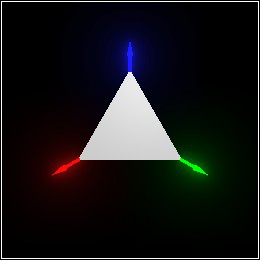> (triangle (vertex (pos 3/4 0 0) #:normal +x) (vertex (pos 0 3/4 0) #:normal +y) (vertex (pos 0 0 3/4) #:normal +z))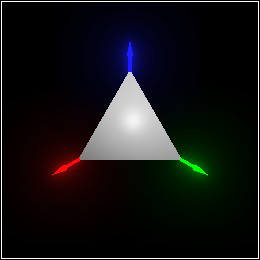> (triangle (vertex (pos 3/4 0 0) #:color (rgba "red")) (vertex (pos 0 3/4 0) #:emitted (emitted "lightgreen" 2.0)) (vertex (pos 0 0 3/4) #:material (material #:specular 1.0 #:roughness 0.1)))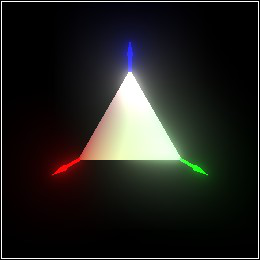procedure

 (quad corner1 corner2 corner3 corner4 [ #:back? back?]) → Pict3D
corner1 : (U Pos Vertex)
corner2 : (U Pos Vertex)
corner3 : (U Pos Vertex)
corner4 : (U Pos Vertex)
back? : Any = #f
Returns a Pict3D containing a quadrilateral with the given corners. The rule for its visibility, and the interpretation of positions and vertices, are the same as for triangle.

A quad’s corners are not required to lie in a plane, so its default normal is a best-fit direction vector computed using Newell’s method.

procedure

position : Pos
color : Emitted = (emitted "white")
r : Real = ((current-light-range) color)
Returns a Pict3D containing a point light source at position, with emitted color color.

Example:
 > (pict3d->bitmap (combine (sphere origin 1) (light (pos 0 1.5 1.5) (emitted "oldlace" 5))))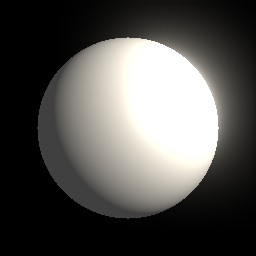A naive rendering algorithm would take time proportional to n·m, where n is the number of objects in a scene and m is the number of lights. Pict3D uses deferred lighting algorithms that take time proportional to n+m.

Deferred lighting algorithms “draw” each light on every fragment of the rendered scene that shows a surface the light might illuminate. An ideal point light illuminates surfaces at any distance, so each ideal point light would have to be drawn on the entire rendered scene. Even with these optimizations, lights tend to take more time to render than solid objects. Try to use only a few thousand well-spaced lights. Adding ideal point lights quickly becomes prohibitively expensive, so Pict3D restricts each light’s effect to a light-specific range r.

Instead of forcing a light’s effect to zero at distance r, Pict3D forces smooth attenuation to zero over a finite range. Forced attenuation starts at distance (/ r 2) and follows a quadratic curve. The quadratic curve’s first derivative matches the ideal’s curve’s first derivative at (/ r 2), and is zero at r (so the overall attenuation is C¹-continuous), which reduces visual artifacts.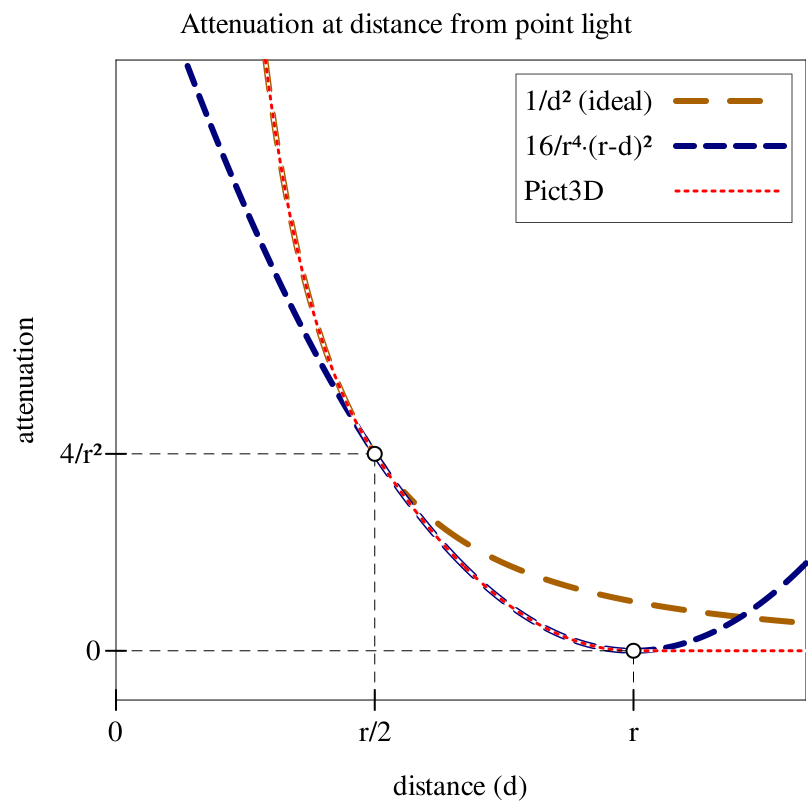With the default value of r, forced attenuation starts when ideal attenuation is 4/40 = 0.1, and ends when ideal attenuation would be 1/40 = 0.025. (See current-light-range.) Thus, a single light’s effect with the default r tends to be hard to distinguish from an ideal light’s effect. Much smaller values of r can result in major discrepancies.

Examples:
 > (pict3d->bitmap (combine (rectangle (pos 0 0 -1) (dir 10 10 1)) (light (pos 0 0 1) (emitted "orange" 1))))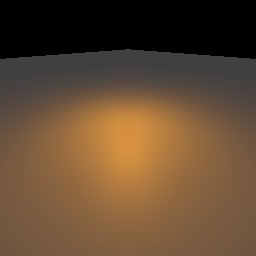> (pict3d->bitmap (combine (rectangle (pos 0 0 -1) (dir 10 10 1)) (light (pos 0 0 1) (emitted "orange" 1) #:range +inf.0)))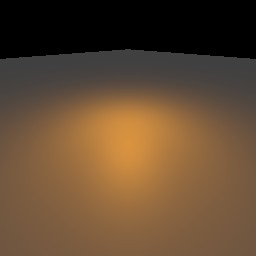> (pict3d->bitmap (combine (rectangle (pos 0 0 -1) (dir 10 10 1)) (light (pos 0 0 1) (emitted "orange" 1) #:range 1.5)))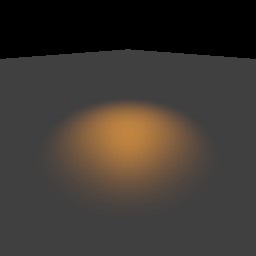In fact, with #:range 1 in the above example, the light has no apparent effect at all.

Small ranges are useful when a light is used for a local visual effect (such as a magical shine on a blade) or when many lights in one area slow down rendering too much.

Large ranges are useful when many weak lights illuminate a surface. Try to choose the smallest large range that gives an effect similar to that of #:range +inf.0.

Examples:
 > (pict3d->bitmap (combine (rectangle (pos 0 0 -1) (dir 5 5 1)) (for*/list ([x  (in-range -5 5.5 0.5)] [y  (in-range -5 5.5 0.5)]) (light (pos x y 1/2) (emitted "orange" 1/100)))))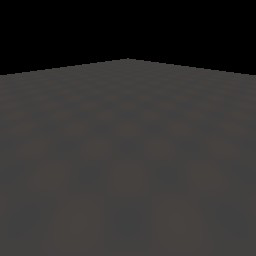> (pict3d->bitmap (combine (rectangle (pos 0 0 -1) (dir 5 5 1)) (for*/list ([x  (in-range -5 5.5 0.5)] [y  (in-range -5 5.5 0.5)]) (light (pos x y 1/2) (emitted "orange" 1/100) #:range (* (sqrt 1/100) 25)))))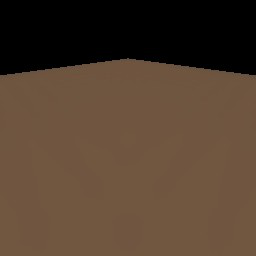The radii argument restricts the light’s area of effect to minimum and maximum fractions of r.

Example:
 > (pict3d->bitmap (combine (rectangle (pos 0 0 -1) 1) (light (pos 0 0 1) (emitted "orange" 10) #:range 30 #:radii (interval 0.044 0.045))))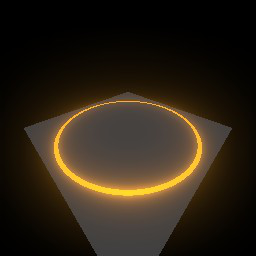Here, the light affects surfaces at distances only in the range [30·0.044,30·0.45] = [1.32,1.35].

Transforming a light may change its initially spherical area of effect to an ellipsoid. This allows lights inside of solid objects to approximate light emission from the objects’ surfaces.

Example:
 > (pict3d->bitmap (combine (rectangle (pos 0 0 -1) (dir 2 2 1)) (transform (combine (with-emitted (emitted "plum" 2) (cylinder origin 1)) (light origin (emitted "plum" 5))) (affine-compose (rotate-z 30) (move-z 1/4) (rotate-x 90) (scale (dir 1/8 1/8 1))))))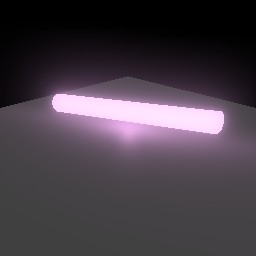Using many smaller, unscaled lights gives a better approximation, and works well for deformed objects.

Example:
 > (pict3d->bitmap (combine (rectangle (pos 0 0 -1) (dir 2 2 1)) (deform (combine (with-emitted (emitted "plum" 2) (tessellate (scale (cylinder origin 1) (dir 1/8 1/8 1)))) (for/list ([z  (in-range 0 17)]) (light (pos 0 0 (- (* 2 (/ z 16)) 1)) (emitted "plum" 1/150)))) (smooth-compose (rotate-z 30) (move-z 1/4) (rotate-x 90) (bend 135 (interval -1 1))))))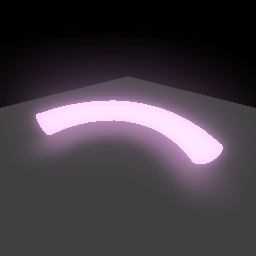procedure

(current-light-range range-fun)  Void
range-fun : (-> Emitted Real)
 = default-light-range
 value = (λ (color) (sqrt (/ (emitted-intensity color) 1/40)))
The default value producer for light’s #:range argument, and its default value. These are provided to make it easy to change every light’s range at once.

The default value is arrived at by solving the ideal attenuation equation l = i/r² for r, where i is (emitted-intensity color) and l = 1/40. That is, it finds the range at which an ideal light’s attenuation is 1/40.

 procedure(sunlight direction [color]) → Pict3D direction : Dir color : Emitted = (emitted "white")
Returns a Pict3D containing an omnipresent, directional light source.

Example:
 > (pict3d->bitmap (combine (sphere origin 1) (sunlight (dir -1 2 0) (emitted "azure" 5))))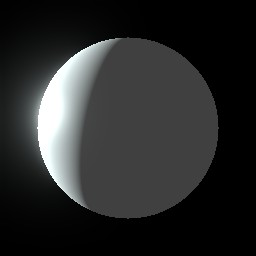procedure

(arrow start end [#:normalize? normalize?])  Pict3D

start : Pos
end : Pos
normalize? : Any = #f
 (arrow start direction [ #:normalize? normalize?]) → Pict3D
start : Pos
direction : Dir
normalize? : Any = #f
Returns a Pict3D containing an arrow drawn from start to end, or from start in the direction direction. When normalize? is non-#f, the arrow has length 1.

See Position and Direction Vectors for examples.